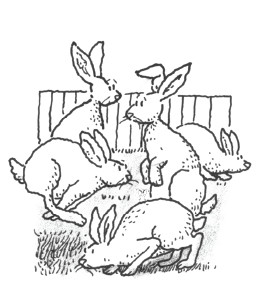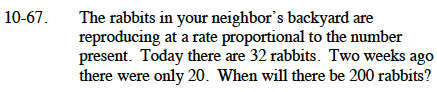### Home > CALC > Chapter 10 > Lesson 10.1.7 > Problem10-67

10-67.

The rabbits in your neighbor's backyard are reproducing at a rate proportional to the number present. Today there are 32 rabbits. Two weeks ago there were only 20. When will there be 200 rabbits? Homework Help ✎If P = rabbit population, then:

Use separation of variables then integrate to obtain:

$P=Ce^{kt}$

Solve for C and k:

Solve for t:

$200=32e^{-0.5\ln(20/32)t}$